Mathman 12 - Practice Parking Sam Bader, Amber Behm, Ben Eastman, Adam Mathiak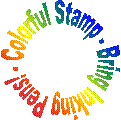Terrain: Plenty of easy walking and lots of driving Difficulty: Depends on math ability Placed by: The Dragon (Mathman) and his Honors Advanced Algebra students of 2004-05 Location: Mequon, WI County: Ozaukee, WI Materials needed: Calculator, Writing Utensil, Rubber Stamp, Inking PensStudents and parents of students should read my introduction to letterboxing before seeking the boxes.

These clues rely heavily on the use of matrices to encode/decode clues.  Go to this page to learn how to do this on a free graphics calculator simulator or to do it by hand.

History of
Mequon

1836 – The first white settlers of Mequon were Yankees from New York state and English, followed by Germans and Irish. The entire area was covered by dense forest. Running through Mequon, the Milwaukee River offered fresh water springs. The word “Mequon” comes from an Indian word “Miguan”, which means “ladle”, because the river in Mequon was shaped like an Indian ladle.

Clues:

Be sure to read through all the clues before looking for the boxes so you know what is expected of you.

Take I-43 to exit 89 (County Highway C). After exiting the freeway, head west on County Highway C to the Mobile gas station on the corner of County Highway C and Port Washington Road. Pull into the gas station and count the number of nozzles at the pumps ______ (A).

Algebraic symbols are used when you do not know what you are talking about.

Leave the gas station heading south on Port Washington Road. Continue on Port Washington Road until Highland Road. Head west on Highland Road, and note the beginning speed limit ______ (B). However, the speed limit later changes to ______ (C). Now pull off somewhere and complete Step One in the "Mathematical Clues" section.

Stay on Highland Road until reaching the first destination ______________________________, which is indicated by your solution to Step One. Pull into the WEST parking lot of this location. Also, find and record the parking lot speed limit ______ (D).

Facing north in the parking lot, head to the open pavilion. Count the number of names on a plaque on one of the pillars. Add four to the total number of names to get ______ (E).

Old mathematicians never die; they just lose some of their functions.

Now walk on the path that runs between the two ponds. Count the number of planks while crossing the bridge ______ (F). After crossing the bridge count the large rocks with engravings on them ______ (G). While sitting on the rock benches complete Steps Two and Three in the "Mathematical Clues" section.

Head towards the other pavilion at the north end of the location. On the east side of the pavilion, there is a baseball field. Record the number written on the center field fence ______ (M).

From the south end of the baseball field (right field corner), walk south ______ (L) paces parallel to the road. To the east is a notable location, and the first box is hidden among three tall pine trees. Carefully find the box, get the stamp, and solve Step Four in the "Mathematical Clues" section to find the next location.

Medicine makes people ill, mathematics make them sad and theology makes them sinful.

(Martin Luther)

Leave this location heading west on Highland Road. At the first stop light, head south on Cedarburg Road for a considerable distance until you get to the next location: ______________________________. This location is on the east side of the road.

At this new location, begin by counting the number of complete pillars on one side of the pavilion ______ (N). Now find a comfortable place to complete Steps Five, Six, Seven, and Eight in the "Mathematical Clues" section.

After exercising the brain, it is time to exercise a well rested body. From the southeast corner of the pavilion walk east (at a bearing 77 degrees) to a white sign off in the distance. Then, walk north (at a bearing of 35 degrees) to a row of sapling trees just before the wooded area on the river. From the westernmost tree, walk ______ (R+2) paces at a bearing of 300 degrees, then ______ (H+5) paces at a bearing of 22 degrees, and finally walk ______ (J+8) paces at a bearing of 116 degrees. The second box is hidden under some loose wood in the hollowed-out end of a fallen trunk in this clump of fallen trees. Carefully find the box, stamp in, and solve Step Nine in the "Mathematical Clues" section to find the next location.

Classification of mathematical problems as linear and nonlinear is like classification of the universe as bananas and non-bananas.

Leave this location heading north on Cedarburg Road. At Mequon Road head east for a considerable distance (who knew Mequon was this big!), and stay on the road even when it makes a ninety degree turn to the south. Although the road changes names three times, the location ______________________________ will be on the east side. (Note: This park is supposed to be open from 7am until sunset, but seems to closed more often due to vandalism.  Hopefully it will be open when you get there!)

After arriving at this new location, head to the playground equipment. Count the number of steps on the large metal slide ______ (T). Now find a place to complete Steps Ten, Eleven, and Twelve in the "Mathematical Clues" section.

After all that work, head to the covered picnic area, and count the support beams on the wooden structure ______ (AE). Next to the structure is a long row of trees to the west. Starting with the southernmost tree, count northward ______ (A divided by 3) trees. In a stump to the west is the third box. Carefully find the box, get the stamp, and solve Steps Thirteen, Fourteen, Fifteen, Sixteen, Seventeen, Eighteen, and Nineteen in the "Mathematical Clues" section to find the next location.

A mathematician is a blind man in a dark room looking for a black cat which isn't there.

Leaving this location, head to the next location ______________________________ using only the piecewise graph. At this final location, head to the back parking lot ("The Back 40") and walk ______ (AG+15) paces from the edge of the parking lot on down the trail to two benches. Walk to the east to find the final box nestled in the crook of a three trunked tree.

Congratulations!

Mathematical Clues:

Step One:

Use the digits of (B) and (C) to form a two by two matrix. Multiply the inverse of this matrix by the following two by six matrix. Decode the numbers to reveal the first location.Step Two:

Find the line that is perpendicular to y = -2x + 52 and passes through the point (7,8). Then find the point where the two lines intersect so that x = (H) and y = (J).

Step Three:

Find the inverse of the following relation:      (173, 1), (216, 2), (332, 4)

Then find a quadratic function, f (x) = (G)x2 + (K)x + (L), that fits the new data points exactly.

Step Four:

Multiply (E) by (H) to form a four digit number, and use it to create a two by two matrix. Multiply the inverse of this matrix by the following two by five matrix. Decode the numbers for the next location.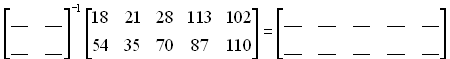Step Five:

Solve the following system of equations. Then combine the solutions to form a three digit number xyz which will be (P).

3x + 18y + 6z   = 141

73y – 699 = -50z – 11x

x = 1

Step Six:

Simplify the following equation and solve for (Q) substituting the value of (P).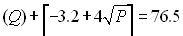(Note: The things that look like brackets are our textbook's symbols for the "rounding-up" operation.  Whatever is inside gets rounded up to the nearest integer.)

Step Seven:

Simplify the following equation and solve for (R) substituting the values of (M) and (N).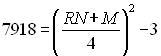Step Eight:

Find the y-intercept, (S), for the following equation: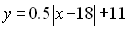Step Nine:

Combine (N) and (P) to form a four digit number, and use it to create a two by two matrix. Multiply the inverse of this matrix by the following two by six matrix. Decode the numbers for the next location.Step Ten:

Solve for (T), (U), and (V) by solving the following system of equations using elimination, substitution, matrices, or another method.

(T) = ?

72(T) + 5(U) – 20(V) = -830

.5(U) + 4(V) = 460

Step Eleven:

Solve for (AA) and (AB) by solving the following system of equations using substitution.

5(AA) + 20(AB) = 1402.5

3(AA) + 19(AB) = 1118

Step Twelve:

Solve for (AC) and (AD) where (AC) is the coefficient and (AD) is the y-intercept.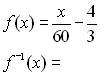Step Thirteen:

Multiply (AB) by (AC) to form a four digit number, and use it to create a two by two matrix. Multiply the inverse of this matrix by the following two by twelve matrix. Decode the numbers for the next location.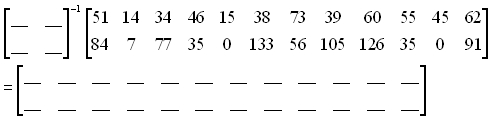Step Fourteen:

Find the positive root, (AG), for the following equation.Step Fifteen:

Find (AH), by finding the slope between ((U), (G)) and ((P), (Q)) leaving the answer as a reduced fraction. Also, find (AJ), by finding the slope between ((P), (Q)) and ((V), (E)) leaving the answer as a reduced fraction.  Also, find (AK), by finding the slope between ((U), (G)) and ((V), (E)) leaving the answer as a reduced fraction.

Step Sixteen:

On a graphics calculator or the graph provided, plot (scatterplot) the following points.

(Set the up graph so that the x-axis goes from zero to one hundred seventy and the y-axis goes from zero to one hundred twenty. Use an appropriate scale. I suggest going by 5's on the provided graph paper.)

((U), (G))

((P), (Q))

((V), (E))

((AA), (AB))

Step Seventeen:

On the same graph, graph the following piecewise equation.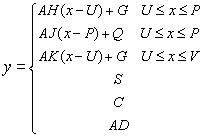Step Eighteen:

Finally, graph the following vertical lines on the graph:

x = (U)             x = (AC)                      x = (L)

Step Nineteen:

If done correctly, this graph should be a map of Mequon so that the x-axis is County Line Road, and the y-axis is Wauwatosa Road. The top edge of the graph is Pioneer Road, and the right edge of the graph is Lake Michigan.  The three points forming a triangle with the line diagonal lines are the three places you've already been to.  The remaining point is the last place you need to head to.Before you set out read the waiver of responsibility and disclaimer.

LbNA Home